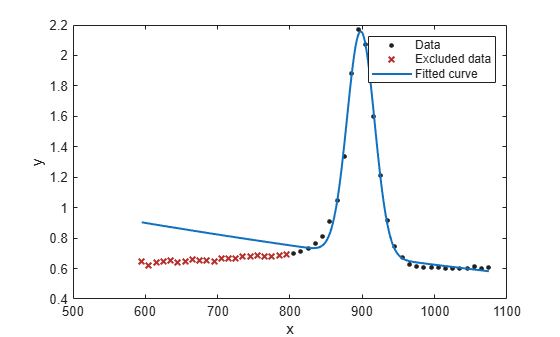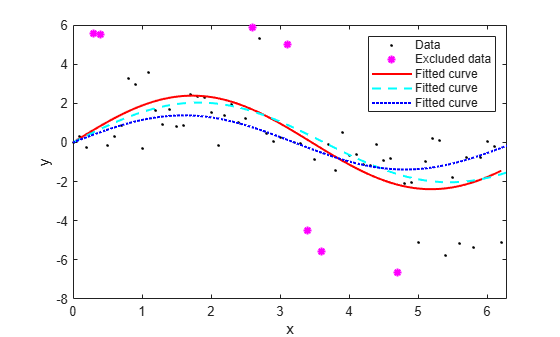Main Content

## Remove Outliers

### Remove Outliers Interactively

To remove outliers in the Curve Fitting app, follow these steps:

1. Select Tools > Exclude Outliers or click the toolbar button.

When you move the mouse cursor to the plot, it changes to a cross-hair to show you are in outlier selection mode.

2. Click a point that you want to exclude in the main plot or residuals plot. Alternatively, click and drag to define a rectangle and remove all enclosed points.

A removed plot point becomes a red cross in the plots. If you have selected, the Curve Fitting app refits the surface without the point. Otherwise, you can click to refit.

3. Repeat for all points you want to exclude.

When removing outliers from surface fits, it can be helpful to display a 2-D residuals plot for examining and removing outliers. With your plot cursor in rotation mode, right-click the plot to select X-Y, X-Z, or Y-Z view.

To replace individual excluded points in the fit, click an excluded point again in Exclude Outliers mode. To replace all excluded points in the fit, right-click and select Clear all exclusions.

In surface plots, to return to rotation mode, click the Exclude outliers toolbar buttonagain to turn off outlier selection mode.

### Exclude Data Ranges

To exclude sections of data by range in the Curve Fitting app, follow these steps:

1. Select Tools > Exclude By Rule.

2. Specify data to exclude. Enter numbers in any of the boxes to define beginning or ending intervals to exclude in the X, Y, or Z data.

3. Press Enter to apply the exclusion rule.

Curve Fitting app displays shaded pink areas on the plots to show excluded ranges. Excluded points become red.

### Remove Outliers Programmatically

This example shows how to remove outliers when curve fitting programmatically, using the 'Exclude' name/value pair argument with the fit or fitoptions functions. You can plot excluded data by supplying an Exclude or outliers argument with the plot function.

Exclude Data Using a Simple Rule

For a simple example, load data and fit a Gaussian, excluding some data with an expression, then plot the fit, data and the excluded points.

```[x, y] = titanium; f1 = fit(x',y','gauss2', 'Exclude', x<800); plot(f1,x,y,x<800)```Exclude Data by Distance from the Model

It can be useful to exclude outliers by distance from the model, using standard deviations. The following example shows how to identify outliers using distance greater than 1.5 standard deviations from the model, and compares with a robust fit which gives lower weight to outliers.

Create a baseline sinusoidal signal:

```xdata = (0:0.1:2*pi)'; y0 = sin(xdata);```

Add noise to the signal with non-constant variance:

```% Response-dependent Gaussian noise gnoise = y0.*randn(size(y0)); % Salt-and-pepper noise spnoise = zeros(size(y0)); p = randperm(length(y0)); sppoints = p(1:round(length(p)/5)); spnoise(sppoints) = 5*sign(y0(sppoints)); ydata = y0 + gnoise + spnoise;```

Fit the noisy data with a baseline sinusoidal model:

```f = fittype('a*sin(b*x)'); fit1 = fit(xdata,ydata,f,'StartPoint',[1 1]);```

Identify "outliers" as points at a distance greater than 1.5 standard deviations from the baseline model, and refit the data with the outliers excluded:

```fdata = feval(fit1,xdata); I = abs(fdata - ydata) > 1.5*std(ydata); outliers = excludedata(xdata,ydata,'indices',I); fit2 = fit(xdata,ydata,f,'StartPoint',[1 1],... 'Exclude',outliers);```

Compare the effect of excluding the outliers with the effect of giving them lower bisquare weight in a robust fit:

`fit3 = fit(xdata,ydata,f,'StartPoint',[1 1],'Robust','on');`

Plot the data, the outliers, and the results of the fits:

```plot(fit1,'r-',xdata,ydata,'k.',outliers,'m*') hold on plot(fit2,'c--') plot(fit3,'b:') xlim([0 2*pi])```## Support

#### Machine Learning Challenges: Choosing the Best Classification Model and Avoiding Overfitting

Download white paper